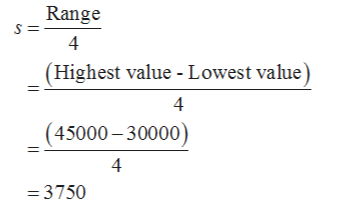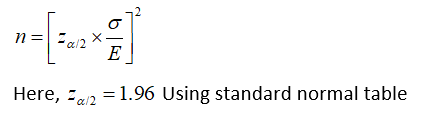# Annual starting salaries for college graduates with degrees in business administration are generally expected to be between \$30,000 and \$45,000. Assume that a 95% confidence interval estimate of the population mean annual starting salary is desired. a. What is the planning value for the population standard deviation?How large a sample should be taken if the desired margin of error isb.\$500?c.\$200?d.\$100?e.Would you recommend trying to obtain the \$100 margin of error? Explain.

Question
1 views

Annual starting salaries for college graduates with degrees in
\$45,000. Assume that a 95% confidence interval estimate of the population mean annual starting salary is desired.
a. What is the planning value for the population standard deviation?

How large a sample should be taken if the desired margin of error is
b.\$500?

c.\$200?

d.\$100?

e.Would you recommend trying to obtain the \$100 margin of error? Explain.

check_circle

Step 1

Given:

Expected highest annual salaries=\$45000

Expected lowest annual salaries=\$30000

Confidence level= 0.95

Therefore,

α=1-0.95=0.05

Step 2

a)The approximate value of the population standard deviationHence, the planning value for population standard deviation is \$3750

Step 3

Now we have to find required sample size n for each margin of error.

Formula:...

### Want to see the full answer?

See Solution

#### Want to see this answer and more?

Solutions are written by subject experts who are available 24/7. Questions are typically answered within 1 hour.*

See Solution
*Response times may vary by subject and question.
Tagged in